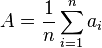## Arithmetic Mean Calculator

 Enter Numbers: 1,2,3,4
 Arithmetic Mean:

In mathematics and statistics, the arithmetic mean is the sum of a collection of numbers divided by the number of numbers in the collection.

### Arithmetic Mean Calculator formula

Data set{a1, a2……an}, then arithmetic mean A isThinkcalculator.com provides you helpful and handy calculator resources.